# Lebesgue integral

2010 Mathematics Subject Classification: Primary: 28A25 [MSN][ZBL]

The most important generalization of the concept of an integral. Let $(X,\mu)$ be a space with a non-negative complete countably-additive measure $\mu$ (cf. Countably-additive set function; Measure space), where $\mu(X)<\infty$. A simple function is a measurable function $g:X\to\mathbb R$ that takes at most a countable set of values: $g(x)=y_n$, $y_n\ne y_k$ for $n\ne k$, if $x\in X_n$, $\bigcup\limits_{n=1}^{\infty}X_n=X$. A simple function $g$ is said to be summable if the series \begin{equation} \sum\limits_{n=1}^{\infty}y_n\mu(X_n) \end{equation} converges absolutely (cf. Absolutely convergent series); the sum of this series is the Lebesgue integral \begin{equation} \int\limits_Xg\,d\mu. \end{equation} A function $f:X\to\mathbb R$ is summable on $X$  if there is a sequence of simple summable functions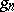uniformly convergent (cf. Uniform convergence) toon a set of full measure, and if the limit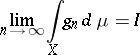is finite. The numberis the Lebesgue integral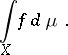This is well-defined: the limitexists and does not depend on the choice of the sequence. If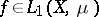, thenis a measurable almost-everywhere finite function on. The Lebesgue integral is a linear non-negative functional on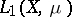with the following properties:

1) ifand ifthen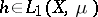and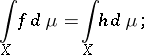2) if, then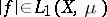and3) if,andis measurable, thenand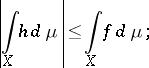4) ifandis measurable, thenandIn the case when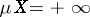and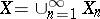,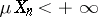, the Lebesgue integral is defined as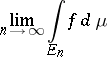under the condition that this limit exists and is finite for any sequence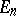such that,,. In this case the properties 1), 2), 3) are preserved, but condition 4) is violated.

For the transition to the limit under the Lebesgue integral sign see Lebesgue theorem.

Ifis a measurable set in, then the Lebesgue integral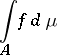is defined either as above, by replacingby, or as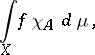where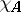is the characteristic function of; these definitions are equivalent. If, thenfor any measurable. If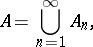ifis measurable for every, if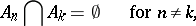and if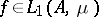, then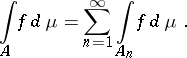Conversely, if under these conditions onone has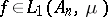for everyand if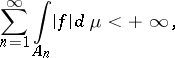thenand the previous equality is true (-additivity of the Lebesgue integral).

The function of sets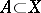given byis absolutely continuous with respect to(cf. Absolute continuity); if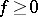, thenis a non-negative measure that is absolutely continuous with respect to. The converse assertion is the Radon–Nikodým theorem.

For functions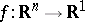the name "Lebesgue integral" is applied to the corresponding functional if the measureis the Lebesgue measure; here, the set of summable functions is denoted simply by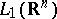, and the integral by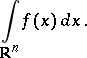For other measures this functional is called a Lebesgue–Stieltjes integral.

If,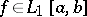and ifis a non-decreasing absolutely continuous function, then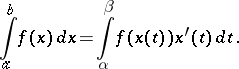If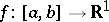,and if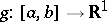is monotone on, then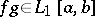and there is a point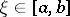such that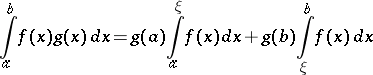(the second mean-value theorem).

In 1902 H. Lebesgue gave (see [Le]) a definition of the integral forand measureequal to the Lebesgue measure. He constructed simple functions that uniformly approximate almost-everywhere on a setof finite measure a measurable non-negative function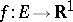, and proved the existence of a common limit (finite or infinite) of the integrals of these simple functions as they tend to. The Lebesgue integral is a basis for various generalizations of the concept of an integral. As N.N. Luzin remarked [Lu], property 2), called absolute integrability, distinguishes the Lebesgue integral for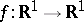from all possible generalized integrals.